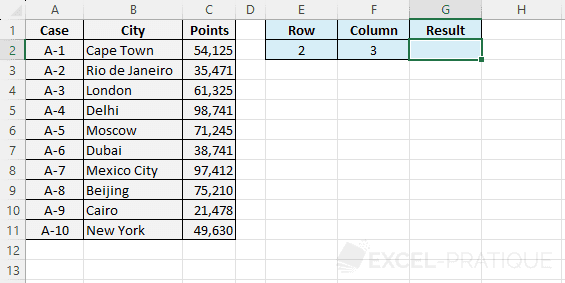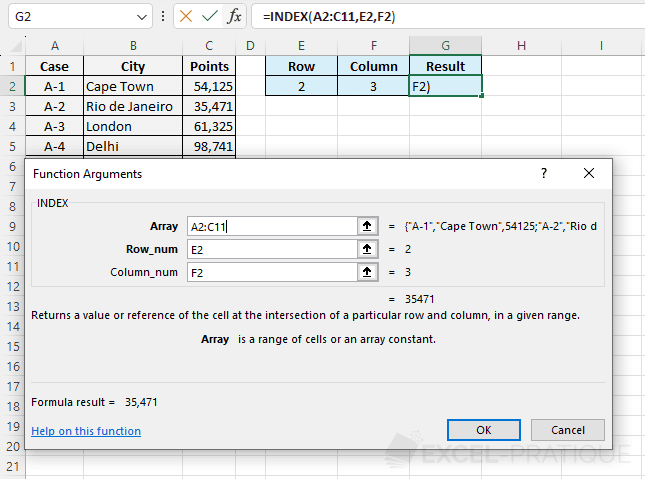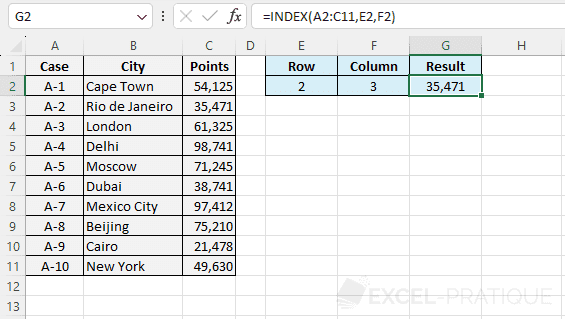# Excel Function: INDEX

The Excel function INDEX searches for a value in an array based on its coordinates.

Usage:

`=INDEX(array, row_no, col_no)`

## Example of use

The goal here is to display a value from the table based on the row and column numbers:Select the INDEX function and enter:

• Array: the table in which the function will perform the search
• Row_num: the row number in Array
• Column_num: the column number in Array
``=INDEX(A2:C11,E2,F2)``The function then displays the value according to its position in the table: